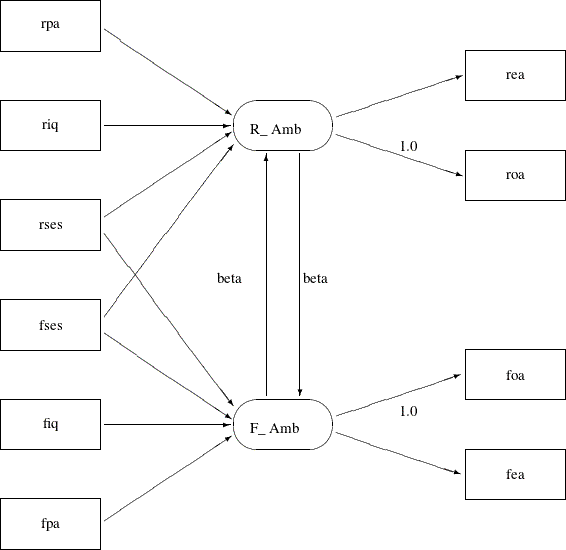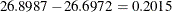### Career Aspiration: Analysis 2

Jöreskog and Sörbom (1988) present more detailed results from a second analysis in which two constraints are imposed:

• The coefficients that connect the latent ambition variables are equal.

• The covariance of the disturbances of the ambition variables is zero.

Applying these constraints to Figure 17.37, you get the path diagram displayed in Figure 17.41.

Figure 17.41: Path Diagram for Career Aspiration : Analysis 2In Figure 17.41, the double-headed path that connected `R_Amb` and `F_Amb` no longer exists. Also, the single-headed paths between `R_Amb` and `F_Amb` are both labeled with `beta`, indicating the required constrained effects in the model. The path diagram in Figure 17.41 is transcribed into the PATH model in the following statements:

```proc calis data=aspire nobs=329;
path
/* structural model of influences */
rpa    ===> R_Amb         ,
riq    ===> R_Amb         ,
rses   ===> R_Amb         ,
fses   ===> R_Amb         ,
rses   ===> F_Amb         ,
fses   ===> F_Amb         ,
fiq    ===> F_Amb         ,
fpa    ===> F_Amb         ,
F_Amb  ===> R_Amb   = beta,
R_Amb  ===> F_Amb   = beta,

/* measurement model for aspiration */
R_Amb  ===> rea         ,
R_Amb  ===> roa     = 1.,
F_Amb  ===> foa     = 1.,
F_Amb  ===> fea     ;
run;
```

The only differences between the current specification and the preceding specification for Analysis 1 are the labeling of two paths with the same parameter `beta` and the deletion of PCOV statement where the covariance of `R_Amb` and `F_Amb` was specified in Analysis 1. The fit summary of the current model is displayed in Figure 17.42, and the estimation results are displayed in Figure 17.43.

Figure 17.42: Career Aspiration Data: Fit Summary for Analysis 2

Fit Summary
Chi-Square 26.8987
Chi-Square DF 17
Pr > Chi-Square 0.0596
Standardized RMR (SRMR) 0.0203
RMSEA Estimate 0.0421
Akaike Information Criterion 102.8987
Schwarz Bayesian Criterion 247.1489
Bentler Comparative Fit Index 0.9880

The model fit chi-square value is 26.8987 (df=17, p=0.0596). The standardized RMSR and the RMSEA are both less than 0.05, while the adjusted GFI and comparative fit index are both bigger than 0.9. All these indicate a good model fit, but how does this model (Analysis 2) compare with that in Analysis 1?

The difference between the chi-square values for Analyses 1 and 2 iswith two degrees of freedom, which is far from significant. This indicates that the restricted model (Analysis 2) fits as well as the unrestricted model (Analysis 1). The AIC is 102.8987, and the SBC is 247.149. Both of these values are smaller than that of Analysis 1 (106.697 for AIC and 258.540 for SBC), and hence they indicate that the current model is a better one.

Figure 17.43: Career Aspiration Data: Estimation Results for Analysis 2

PATH List
Path Parameter Estimate Standard
Error
t Value
rpa ===> R_Amb _Parm01 0.16367 0.03872 4.22740
riq ===> R_Amb _Parm02 0.25395 0.04186 6.06726
rses ===> R_Amb _Parm03 0.22115 0.04187 5.28218
fses ===> R_Amb _Parm04 0.07728 0.04149 1.86264
rses ===> F_Amb _Parm05 0.06840 0.03868 1.76809
fses ===> F_Amb _Parm06 0.21839 0.03948 5.53198
fiq ===> F_Amb _Parm07 0.33063 0.04116 8.03314
fpa ===> F_Amb _Parm08 0.15204 0.03636 4.18169
F_Amb ===> R_Amb beta 0.18007 0.03912 4.60305
R_Amb ===> F_Amb beta 0.18007 0.03912 4.60305
R_Amb ===> rea _Parm09 1.06097 0.08921 11.89233
R_Amb ===> roa   1.00000
F_Amb ===> foa   1.00000
F_Amb ===> fea _Parm10 1.07359 0.08063 13.31498

Variance Parameters
Variance
Type
Variable Parameter Estimate Standard
Error
t Value
Exogenous riq _Add01 1.00000 0.07809 12.80625
Error roa _Add07 0.41205 0.05103 8.07403

Covariances Among Exogenous Variables
Var1 Var2 Parameter Estimate Standard
Error
t Value
rpa riq _Add13 0.18390 0.05614 3.27564
rses riq _Add14 0.22200 0.05656 3.92503
rses rpa _Add15 0.04890 0.05528 0.88456
fiq riq _Add16 0.33550 0.05824 5.76060
fiq rpa _Add17 0.07820 0.05538 1.41195
fiq rses _Add18 0.23020 0.05666 4.06284
fpa riq _Add19 0.10210 0.05550 1.83955
fpa rpa _Add20 0.11470 0.05558 2.06377
fpa rses _Add21 0.09310 0.05545 1.67885
fpa fiq _Add22 0.20870 0.05641 3.70000
fses riq _Add23 0.18610 0.05616 3.31352
fses rpa _Add24 0.01860 0.05523 0.33680
fses rses _Add25 0.27070 0.05720 4.73226
fses fiq _Add26 0.29500 0.05757 5.12435
fses fpa _Add27 -0.04380 0.05527 -0.79249

Like Analysis 1, the same two paths in the current analysis are not significant. That is, `fses` does not seem to be a good indicator of a respondent’s ambition `R_Amb`, and `rses` does not seem to be a good indicator of a friend’s ambition `F_Amb`. The t values are 1.862 and 1.768, respectively.